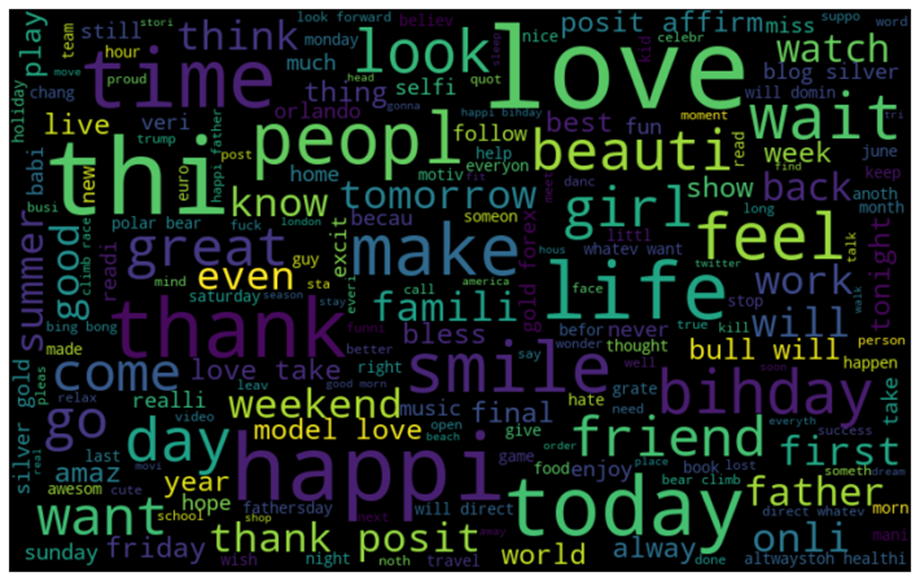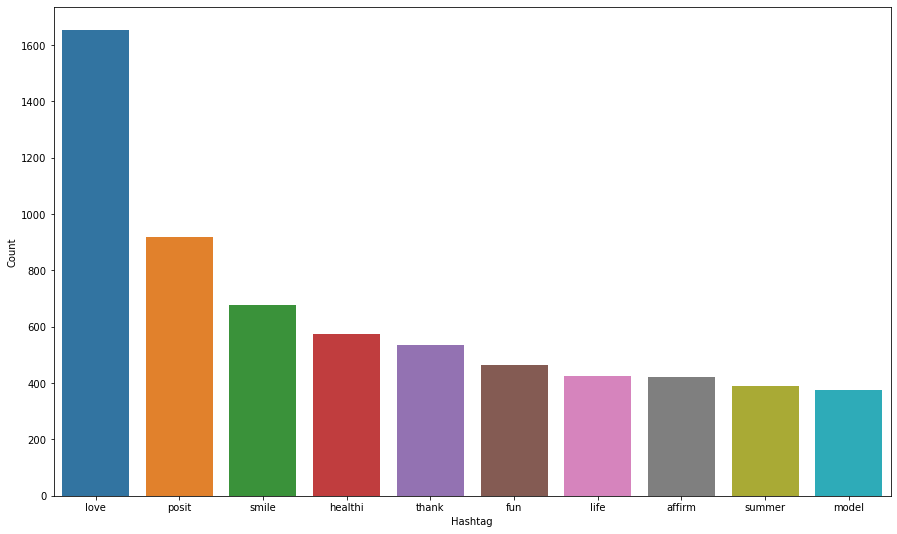top of page
• Hackers Realm

# Twitter Sentiment Analysis using Python (NLP) | Machine Learning Project Tutorial

Updated: Jun 4

Unleash the power of Twitter sentiment analysis using Python! In this comprehensive tutorial, dive into natural language processing (NLP) and machine learning to extract insights from tweets. Explore techniques to preprocess text data, build sentiment classification models, and evaluate their performance. Gain hands-on experience with popular Python libraries and learn how to apply NLP techniques to real-world projects. Join this tutorial to master the art of Twitter sentiment analysis and unlock valuable insights from social media data. #TwitterSentimentAnalysis #Python #NLP #MachineLearning #TextClassification #SentimentAnalysisIn this project tutorial, we are going to analyze and classify tweets from the dataset using a classifying model and visualize the frequent words using plot graphs.

You can watch the step by step explanation video tutorial down below

## Dataset Information

The objective of this task is to detect hate speech in tweets. For the sake of simplicity, we say a tweet contains hate speech if it has a racist or sexist sentiment associated with it. So, the task is to classify racist or sexist tweets from other tweets.

Formally, given a training sample of tweets and labels, where label '1' denotes the tweet is racist/sexist and label '0' denotes the tweet is not racist/sexist, your objective is to predict the labels on the test dataset.

For training the models, we provide a labelled dataset of 31,962 tweets. The dataset is provided in the form of a csv file with each line storing a tweet id, its label and the tweet.

In this analysis we’re going to process text based data, machines can’t understand text-oriented data so we’ll convert the text to vectors and proceed further.

## Import modules

```import pandas as pd
import numpy as np
import matplotlib.pyplot as plt
import seaborn as sns
import re
import string
import nltk
import warnings
%matplotlib inline

warnings.filterwarnings('ignore')```
• pandas - used to perform data manipulation and analysis

• numpy - used to perform a wide variety of mathematical operations on arrays

• matplotlib - used for data visualization and graphical plotting

• seaborn - built on top of matplotlib with similar functionalities

• re – used as a regular expression to find particular patterns and process it

• string – used to obtain information in the string and manipulate the string overall

• nltk – a natural language processing toolkit module associated in anaconda

• warnings - to manipulate warnings details

• %matplotlib - to enable the inline plotting

filterwarnings('ignore') is to ignore the warnings thrown by the modules (gives clean results)

```df = pd.read_csv('Twitter Sentiments.csv')• df.head() displays the 5 first rows from the dataframe

• Zero (0) indicates it’s a positive sentiment.

• One (1) indicates it’s a negative sentiment (racist/sexist).

```# datatype info
df.info()```• The 'tweet' column is an object which will be processed as a string passing the tweets listed above in the pre-processing step.

## Preprocessing the dataset

```# removes pattern in the input text
def remove_pattern(input_txt, pattern):
r = re.findall(pattern, input_txt)
for word in r:
input_txt = re.sub(word, "", input_txt)
return input_txt```
• This function works to remove certain patterns in the text for preprocessing

`df.head()````# remove twitter handles (@user)
df['clean_tweet'] = np.vectorize(remove_pattern)(df['tweet'], "@[\w]*")```
`df.head()`• "@[\w]*" is the twitter handle pattern to remove in the text for preprocessing

```# remove special characters, numbers and punctuations
df['clean_tweet'] = df['clean_tweet'].str.replace("[^a-zA-Z#]", " ")• [^a-zA-Z#] is the parameter to remove all special characters, numbers and punctuations

```# remove short words
df['clean_tweet'] = df['clean_tweet'].apply(lambda x: " ".join([w for w in x.split() if len(w)>3]))Dataset after Cleaning
• Process to remove shorter words less than 3 characters long.

```# individual words considered as tokensTokenized Dataset
• Individual words separated as tokens to facilitate further processing as strings

```# stem the words
from nltk.stem.porter import PorterStemmerstemmer = PorterStemmer()

tokenized_tweet = tokenized_tweet.apply(lambda sentence: [stemmer.stem(word) for word in sentence])Dataset after Stemming
• Stemmer.stem() converts certain words into its simpler version.

```# combine words into single sentence
for i in range(len(tokenized_tweet)):
tokenized_tweet[i] = " ".join(tokenized_tweet[i])

df['clean_tweet'] = tokenized_tweet• Combining the tokenized words into a sentence

## Exploratory Data Analysis

In Exploratory Data Analysis (EDA), we will visualize the data with different kinds of plots for inference. It is helpful to find some patterns (or) relations within the data

`!pip install wordcloud`
```Collecting wordcloud
Requirement already satisfied: pillow in c:\programdata\anaconda3\lib\site-packages (from wordcloud) (7.2.0)
Requirement already satisfied: numpy>=1.6.1 in c:\programdata\anaconda3\lib\site-packages (from wordcloud) (1.18.5)
Requirement already satisfied: matplotlib in c:\programdata\anaconda3\lib\site-packages (from wordcloud) (3.2.2)
Requirement already satisfied: python-dateutil>=2.1 in c:\programdata\anaconda3\lib\site-packages (from matplotlib->wordcloud) (2.8.1)
Requirement already satisfied: cycler>=0.10 in c:\programdata\anaconda3\lib\site-packages (from matplotlib->wordcloud) (0.10.0)
Requirement already satisfied: pyparsing!=2.0.4,!=2.1.2,!=2.1.6,>=2.0.1 in c:\programdata\anaconda3\lib\site-packages (from matplotlib->wordcloud) (2.4.7)
Requirement already satisfied: kiwisolver>=1.0.1 in c:\programdata\anaconda3\lib\site-packages (from matplotlib->wordcloud) (1.2.0)
Requirement already satisfied: six>=1.5 in c:\programdata\anaconda3\lib\site-packages (from python-dateutil>=2.1->matplotlib->wordcloud) (1.15.0)
Installing collected packages: wordcloud
Successfully installed wordcloud-1.8.1```
• Necessary installation process to use the wordcloud

```# visualize the frequent words
all_words = " ".join([sentence for sentence in df['clean_tweet']])

from wordcloud import WordCloud
wordcloud = WordCloud(width=800, height=500, random_state=42, max_font_size=100).generate(all_words)

# plot the graph
plt.figure(figsize=(15,8))
plt.imshow(wordcloud, interpolation='bilinear')
plt.axis('off')
plt.show()```Word Cloud of Frequent Words
• Filtering all frequent words from the data to plot graph using the word cloud

• The plot displaying many positive words and a few negative words

```# frequent words visualization for +ve
all_words = " ".join([sentence for sentence in df['clean_tweet'][df['label']==0]])

wordcloud = WordCloud(width=800, height=500, random_state=42, max_font_size=100).generate(all_words)

# plot the graph
plt.figure(figsize=(15,8))
plt.imshow(wordcloud, interpolation='bilinear')
plt.axis('off')
plt.show()```Word Cloud of Frequent Words for Positive Sentiment
• Filtering more frequent positive words adding a new parameter [df['label']==0]]) indicating positive sentiments

• Comparing with the previous plot graph, there’s more positive words

```# frequent words visualization for -ve
all_words = " ".join([sentence for sentence in df['clean_tweet'][df['label']==1]])

wordcloud = WordCloud(width=800, height=500, random_state=42, max_font_size=100).generate(all_words)

# plot the graph
plt.figure(figsize=(15,8))
plt.imshow(wordcloud, interpolation='bilinear')
plt.axis('off')
plt.show()```Word Cloud of Frequent Words for Negative Sentiment
• For the negative sentiment it’s exactly the same code but changing the value of label to one (1), filtering racist/sexist words used.

```# extract the hashtag
def hashtag_extract(tweets):
hashtags = []
# loop words in the tweet
for tweet in tweets:
ht = re.findall(r"#(\w+)", tweet)
hashtags.append(ht)
return hashtags```
• Extraction of all racist and non-racist hashtag content in the tweets, returning a list of hashtags.

```# extract hashtags from non-racist/sexist tweets
ht_positive = hashtag_extract(df['clean_tweet'][df['label']==0])```
• Extraction of hashtags from positive tweets

```# extract hashtags from racist/sexist tweets
ht_negative = hashtag_extract(df['clean_tweet'][df['label']==1])```
• Extraction of hashtags from negative tweets

`ht_positive[:5]`

[['run'], ['lyft', 'disapoint', 'getthank'], [], ['model'], ['motiv']]

• Viewing the list of the extracted positive hashtags, in this example we are listing five for a simple view.

```# unnest list
ht_positive = sum(ht_positive, [])
ht_negative = sum(ht_negative, [])```
• Filtering and cleaning the words in the sentence for a better visualization and processing

`ht_positive[:5]`

['run', 'lyft', 'disapoint', 'getthank', 'model']

• Listing the words to view the results, now it can be processed more efficiently.

```freq = nltk.FreqDist(ht_positive)
d = pd.DataFrame({'Hashtag': list(freq.keys()),
'Count': list(freq.values())})Hashtags
• Conversion of the dictionary into a dataframe to list positive hashtags with count

```# select top 10 hashtags
d = d.nlargest(columns='Count', n=10)
plt.figure(figsize=(15,9))
sns.barplot(data=d, x='Hashtag', y='Count')
plt.show()```Top 10 Positive Hashtags
• Visualization through a bar graph for top ten positive hashtags with high frequency

```freq = nltk.FreqDist(ht_negative)
d = pd.DataFrame({'Hashtag': list(freq.keys()),
'Count': list(freq.values())})• Conversion of the dictionary into a dataframe to list negative hashtags with count

```# select top 10 hashtags
d = d.nlargest(columns='Count', n=10)
plt.figure(figsize=(15,9))
sns.barplot(data=d, x='Hashtag', y='Count')
plt.show()```Top 10 Negative Hashtags
• Visualization through a bar graph for top ten negative hashtags with high frequency.

## Input Split

The Input Split is a pre-process step for feature selection or feature extraction of the words in order to convert them into vectors for the machine to understand.

```# feature extraction
from sklearn.feature_extraction.text import CountVectorizer
bow_vectorizer = CountVectorizer(max_df=0.90, min_df=2, max_features=1000, stop_words='english')
bow = bow_vectorizer.fit_transform(df['clean_tweet'])```
• Extraction of the data into vectors for training and testing

```from sklearn.model_selection import train_test_split
x_train, x_test, y_train, y_test = train_test_split(bow, df['label'], random_state=42, test_size=0.25)```
• Splitting the data for training and testing with test size of 25%

## Model Training

For this exercise the Logistic Regression model is used, other models may be used by your preference

```from sklearn.linear_model import LogisticRegression
from sklearn.metrics import f1_score, accuracy_score```
```# training
model = LogisticRegression()
model.fit(x_train, y_train)```
```# testing
pred = model.predict(x_test)
f1_score(y_test, pred)```

0.49763033175355453

`accuracy_score(y_test,pred)`

0.9469403078463271

• f1_score() and accuracy_score() gives the performance metrics of the model for the test data.

```# use probability to get output
pred_prob = model.predict_proba(x_test)
pred = pred_prob[:, 1] >= 0.3
pred = pred.astype(np.int)

f1_score(y_test, pred)```

0.5545722713864307

• Predict probability feature to receive a output in probability value

• pred_prob[: , 1] >= 0.3 if result is greater than 30 percent it will assign 1, else it will assign 0

• pred.astype(np.int) assign the value to an integer

`accuracy_score(y_test,pred)`

0.9433112251282693

• The scores have been improved by using the probability values with threshold

`pred_prob >= 0.3`

False

## Final Thoughts

• Machines can’t process text-based data, so we have to convert to numerical form in order to process the data.

• Simplifying and filtering text can achieve cleaner data to process, giving better results.

• You may use different machine learning models of your preference for comparison.

In this project tutorial, we have explored the Twitter Sentiment Analysis dataset as a classification machine learning project. The data has been preprocessed and explored using different plots. We have classified a tweet as a negative sentiment or a positive sentiment and view the frequent use of keywords that are present in the dataset.

Get the project notebook from here